#### Sampford’s PPS Method

Sampford’s method (METHOD=PPS_SAMPFORD) is an extension of Brewer’s method that selects more than two units from each stratum, with probability proportional to size and without replacement. The selection probability for unit i in stratum h equals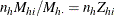. (Because selection probabilities cannot exceed 1, the relative size for each unit,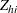, must not exceed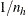.)

Sampford’s method first selects a unit from stratum h with probability. Then subsequent units are selected with probability proportional to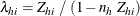and with replacement. If the same unit appears more than once in the sample of size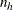, then Sampford’s algorithm rejects that sample and selects a new sample. The sample is accepted if it containsdistinct units.

If you specify the JTPROBS option, PROC SURVEYSELECT computes the joint selection probabilities for all pairs of selected units in each stratum. The joint selection probability for units i and j in stratum h equals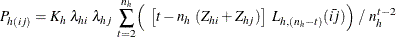where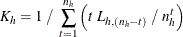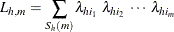and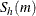denotes all possible samples of size m, for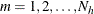. The sum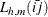is defined similarly to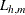but sums over all possible samples of size m that do not include units i and j. See Cochran (1977, pp. 262–263) and Sampford (1967) for details.